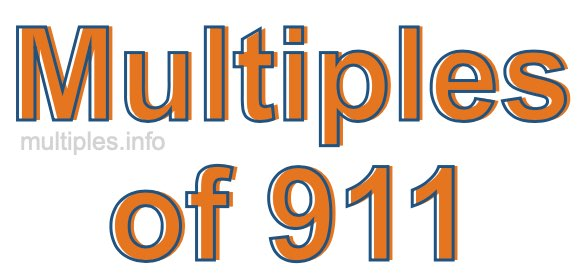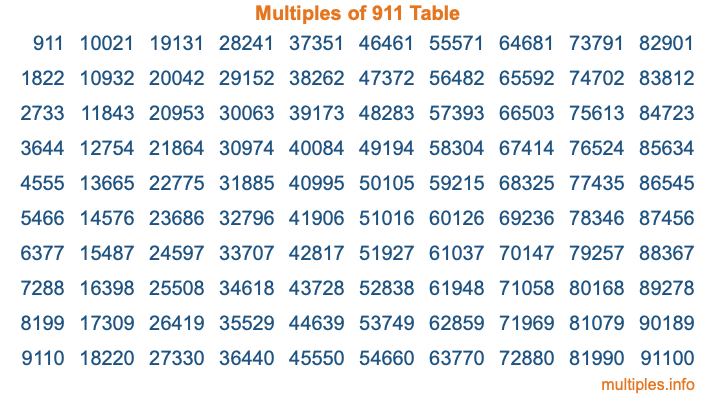Multiples of 911Welcome to the Multiples of 911 page. Here we will first teach you everything you will ever need to know about the multiples of 911, and then give you a study guide summary of everything we taught you to make sure you remember it all. Use this page to look up facts and learn information about the multiples of 911. This page will make you a multiples of nine hundred eleven expert!

Definition of Multiples of 911
Multiples of 911 are all the numbers that when divided by 911 equal an integer. Each of the multiples of 911 are called a multiple. A multiple of 911 is created by multiplying 911 by an integer.

Therefore, to create a list of multiples of 911, you start with 1 multiplied by 911, then 2 multiplied by 911, then 3 multiplied by 911, and so on for as long as you want. Thus, the list of the first five multiples of 911 is 911, 1822, 2733, 3644, and 4555. To see a larger list of multiples of 911, see the printable image of Multiples of 911 further down on this page. We also have a category where you can choose any nth multiple of 911.

Multiples of 911 Checker
The Multiples of 911 Checker below checks to see if any number of your choice is a multiple of 911. In other words, it checks to see if there is any number (integer) that when multiplied by 911 will equal your number. To do that, we divide your number by 911. If the the quotient is an integer, then your number is a multiple of 911.

Is  a multiple of 911?

Least Common Multiple of 911 and ...
A Least Common Multiple (LCM) is the lowest multiple that two or more numbers have in common. This is also called the smallest common multiple or lowest common multiple and is useful to know when you are adding our subtracting fractions. Enter one or more numbers below (911 is already entered) to find the LCM.

Check out our LCM Calculator if you need more details about the Least Common Multiple or if you need the LCM for different numbers for adding and subtraction fractions.

nth Multiple of 911
As we stated above, 911 is the first multiple of 911, 1822 is the second multiple of 911, 2733 is the third multiple of 911, and so on. Enter a number below to find the nth multiple of 911.

th multiple of 911

Multiples of 911 vs Factors of 911
911 is a multiple of 911 and a factor of 911, but that is where the similarities end. All postive multiples of 911 are 911 or greater than 911. All positive factors of 911 are 911 or less than 911.

Below is the beginning list of multiples of 911 and the factors of 911 so you can compare:

Multiples of 911: 911, 1822, 2733, 3644, 4555, etc.

Factors of 911: 1, 911

As you can see, the multiples of 911 are all the numbers that you can divide by 911 to get a whole number. The factors of 911, on the other hand, are all the whole numbers that you can multiply by another whole number to get 911.

It's also interesting to note that if a number (x) is a factor of 911, then 911 will also be a multiple of that number (x).

Multiples of 911 vs Divisors of 911
The divisors of 911 are all the integers that 911 can be divided by evenly. Below is a list of the divisors of 911.

Divisors of 911: 1, 911

The interesting thing to note here is that if you take any multiple of 911 and divide it by a divisor of 911, you will see that the quotient is an integer.

Multiples of 911 Table
Below is an image of the first 100 multiples of 911 in a table. The table is in chronological order, column by column. The first column has the first ten multiples of 911, the second column has the next ten multiples of 911, and so on.The Multiples of 911 Table is also referred to as the 911 Times Table or Times Table of 911. You are welcome to print out our table for your studies.

Negative Multiples of 911
Although not often discussed or needed in math, it is worth mentioning that you can make a list of negative multiples of 911 by multiplying 911 by -1, then by -2, then by -3, and so on, to get the following list of negative multiples of 911:

-911, -1822, -2733, -3644, -4555, etc.

Multiples of 911 Summary
Below is a summary of important Multiples of 911 facts that we have discussed on this page. To retain the knowledge on this page, we recommend that you read through the summary and explain to yourself or a study partner why they hold true.

There are an infinite number of multiples of 911.

A multiple of 911 divided by 911 will equal a whole number.

911 divided by a factor of 911 equals a divisor of 911.

The nth multiple of 911 is n times 911.

The largest factor of 911 is equal to the first positive multiple of 911.

911 is a multiple of every factor of 911.

911 is a multiple of 911.

A multiple of 911 divided by a divisor of 911 equals an integer.

911 divided by a divisor of 911 equals a factor of 911.

Any integer times 911 will equal a multiple of 911.

Multiples of a Number
Here you can get the multiples of another number, all with the same attention to detail as we did for multiples of 911 on this page.

Multiples of
Multiples of 912
Did you find our page about multiples of nine hundred eleven educational? Do you want more knowledge? Check out the multiples of the next number on our list!